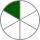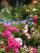# Fraction calculator

The calculator performs basic and advanced operations with fractions, expressions with fractions combined with integers, decimals, and mixed numbers. It also shows detailed step-by-step information about the fraction calculation procedure. Solve problems with two, three, or more fractions and numbers in one expression.

## Result:

### 4/7 + 1/2 = 15/14 = 1 1/14 ≅ 1.0714286

Spelled result in words is fifteen fourteenths (or one and one fourteenth).

### How do you solve fractions step by step?

1. Add: 4/7 + 1/2 = 4 · 2/7 · 2 + 1 · 7/2 · 7 = 8/14 + 7/14 = 8 + 7/14 = 15/14
For adding, subtracting, and comparing fractions, it is suitable to adjust both fractions to a common (equal, identical) denominator. The common denominator you can calculate as the least common multiple of both denominators - LCM(7, 2) = 14. In practice, it is enough to find the common denominator (not necessarily the lowest) by multiplying the denominators: 7 × 2 = 14. In the following intermediate step, it cannot further simplify the fraction result by canceling.
In other words - four sevenths plus one half = fifteen fourteenths.

#### Rules for expressions with fractions:

Fractions - simply use a forward slash between the numerator and denominator, i.e., for five-hundredths, enter 5/100. If you are using mixed numbers, be sure to leave a single space between the whole and fraction part.
The slash separates the numerator (number above a fraction line) and denominator (number below).

Mixed numerals (mixed fractions or mixed numbers) write as integer separated by one space and fraction i.e., 1 2/3 (having the same sign). An example of a negative mixed fraction: -5 1/2.
Because slash is both signs for fraction line and division, we recommended use colon (:) as the operator of division fractions i.e., 1/2 : 3.

Decimals (decimal numbers) enter with a decimal point . and they are automatically converted to fractions - i.e. 1.45.

The colon : and slash / is the symbol of division. Can be used to divide mixed numbers 1 2/3 : 4 3/8 or can be used for write complex fractions i.e. 1/2 : 1/3.
An asterisk * or × is the symbol for multiplication.
Plus + is addition, minus sign - is subtraction and ()[] is mathematical parentheses.
The exponentiation/power symbol is ^ - for example: (7/8-4/5)^2 = (7/8-4/5)2

#### Examples:

subtracting fractions: 2/3 - 1/2
multiplying fractions: 7/8 * 3/9
dividing Fractions: 1/2 : 3/4
exponentiation of fraction: 3/5^3
fractional exponents: 16 ^ 1/2
adding fractions and mixed numbers: 8/5 + 6 2/7
dividing integer and fraction: 5 ÷ 1/2
complex fractions: 5/8 : 2 2/3
decimal to fraction: 0.625
Fraction to Decimal: 1/4
Fraction to Percent: 1/8 %
comparing fractions: 1/4 2/3
multiplying a fraction by a whole number: 6 * 3/4
square root of a fraction: sqrt(1/16)
reducing or simplifying the fraction (simplification) - dividing the numerator and denominator of a fraction by the same non-zero number - equivalent fraction: 4/22
expression with brackets: 1/3 * (1/2 - 3 3/8)
compound fraction: 3/4 of 5/7
fractions multiple: 2/3 of 3/5
divide to find the quotient: 3/5 ÷ 2/3

The calculator follows well-known rules for order of operations. The most common mnemonics for remembering this order of operations are:
PEMDAS - Parentheses, Exponents, Multiplication, Division, Addition, Subtraction.
BEDMAS - Brackets, Exponents, Division, Multiplication, Addition, Subtraction
BODMAS - Brackets, Of or Order, Division, Multiplication, Addition, Subtraction.
GEMDAS - Grouping Symbols - brackets (){}, Exponents, Multiplication, Division, Addition, Subtraction.
Be careful, always do multiplication and division before addition and subtraction. Some operators (+ and -) and (* and /) has the same priority and then must evaluate from left to right.

## Fractions in word problems:

• Unknown numberI think the number - its sixth is 3 smaller than its third.
• 3 children3 children had to divide 4 pounds is candy. How much candy did each child get?
• UN 1If we add to an unknown number his quarter, we get 210. Identify unknown number.
• Tank 11When 150 litres has been drawn from a tank it is 3/8 full, how many litres will the tank hold?
• Find the 11Find the quotient of 229.12 and 12.32
• SummerjobMiloš saved a quarter of the money from the summerjob, bought a winter jacket for half, bought gifts for his parents and siblings for a sixth, and still had CZK 400 left. How much did Miloš earn?
• Class 8.AThree quarters of class 8.A went skiing. Of those who remained at home one third was ill and the remaining six were on math olympic. How many students have class 8.A?
• CupcakesIn a bowl was some cupcakes. Janka ate one third and Danka ate one quarter of cupcakes. a) How many of cookies ate together? b) How many cookies remain in a bowl? Write the results as a decimal number and in notepad also as a fraction.
• PlayingHow long have we trained on the pitch when we know that the warm-up took 10 minutes, we trained passes for one-third of the time and we played football half the time?
• One sixthHow many sixths are two thirds?
• RosesOn the large rosary were a third white, half red, yellow quarter, and six pinks. How many roses were in the rosary?
• Square metal sheetWe cut out four squares of 300 mm side from a square sheet metal plate with a side of 0,7 m. Express the fraction and the percentage of waste from the square metal sheet.
• Cube, cuboid, and sphereVolumes of a cube and a cuboid are in ratio 3: 2. Volumes of sphere and cuboid are in ratio 1: 3. At what rate are the volumes of cube, cuboid, and sphere?